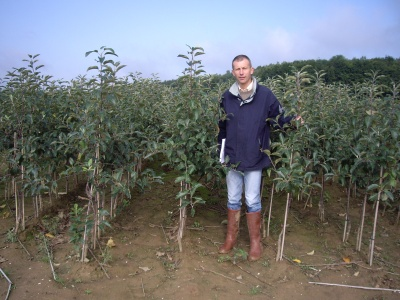FRUIT TREESMost of our fruit trees are produced by Dalival, based in Northern France, at Villers-Cotterets (approx 50 miles NE of Paris). Dalival also has nurseries in other parts of France, and works closely with some nurseries elsewhere in Europe. The nursery at Villers-Cotterets specialises in apple and pear tree production, with much of the cherry, plum, peach, nectarine, apricot, and rootstock production coming from its other nurseries. Commercial growers are able to visit the main nursery by arrangement. For further information please visit www.dalival.com, or contact J R BREACH on 01580 891756

SELECTA®

In order to produce more evenly-coloured fruits throughout the orchard, Dalival have developed a new method of selecting budwood called SELECTA®. Every year, the crop on each mother tree is checked for colour and type. Those trees which do not have the required characteristics are eliminated, with only the remaining trees being used for further propagation. This scheme is associated with the French Certification Scheme.

NEW ORCHARDS - TREES REQUIRED PER HECTARE (ACRE)

There are 10,000 square metres in one hectare, and 43560 square feet in one acre. One hectare equals 2.471 acres.

SINGLE ROW SYSTEMS

For single row systems, to calculate the number of trees required to plant 1 hectare (or 1 acre), divide into 10,000 (or 43560 for acres) the product of the tree spacing (down the row) in metres (or feet and inches) by the row width in metres (or feet and inches).

 Tree Spacing Alley Width 0.75m   (2'6") x 3.00m   (9'10") = 4444/hectare (1798 acre) 1.00m   (3'3") x 3.00m   (9'10") = 3333/hectare (1349 acre) 1.25m   (4'1") x 3.00m   (9'10") = 2666/hectare (1079 acre) 1.50m   (4'11") x 3.00m   (9'10") = 2222/hectare (899 acre) 1.75m   (5'9") x 3.00m   (9'10") = 1905/hectare (771 acre) 2.00m   (6'7") x 3.00m   (9'10") = 1667/hectare (674 acre) 0.75m   (2'6") x 3.50m   (11'6") = 3810/hectare (1542 acre) 1.00m   (3'3") x 3.50m   (11'6") = 2857/hectare (1156 acre) 1.25m   (4'1") x 3.50m   (11'6") = 2286/hectare (925 acre) 1.50m   (4'11") x 3.50m   (11'6") = 1905/hectare (771 acre) 1.75m   (5'9") x 3.50m   (11'6") = 1633/hectare (661 acre) 2.00m   (6'7") x 3.50m   (11'6") = 1429/hectare (578 acre) 0.75m   (2'6") x 3.75m   (12'4") = 3556/hectare (1439 acre) 1.00m   (3'3") x 3.75m   (12'4") = 2667/hectare (1079 acre) 1.25m   (4'1") x 3.75m   (12'4") = 2133/hectare (863 acre) 1.50m   (4'11") x 3.75m   (12'4") = 1778/hectare (719 acre) 1.75m   (5'9") x 3.75m   (12'4") = 1524/hectare (617 acre) 2.00m   (6'7") x 3.75m   (12'4") = 1333/hectare (540 acre) 0.75m   (2'6") x 4.00m   (13'1") = 3333/hectare (1349 acre) 1.00m   (3'3") x 4.00m   (13'1") = 2500/hectare (1012 acre) 1.25m   (4'4") x 4.00m   (13'1") = 2000/hectare (891 acre) 1.50m   (4'11") x 4.00m   (13'1") = 1667/hectare (674 acre) 1.75m   (5'9") x 4.00m   (13'1") = 1429/hectare (578 acre) 2.00m   (6'7") x 4.00m   (13'1") = 1250/hectare (506 acre)

DOUBLE ROW SYSTEMS

For double row systems, to calculate the number of trees required to plant 1 hectare (acre) divide into 10,000 (43560) the product of the tree spacing (down the rows) in metres (feet and inches) by the total of the alley width in metres (feet and inches) plus the row width (distance between the 2 rows in the bed) and then multiply the number obtained by two.

 Tree spacing Row spacing Alley Width 2m x 1.5m x 4m = 1818/hectare